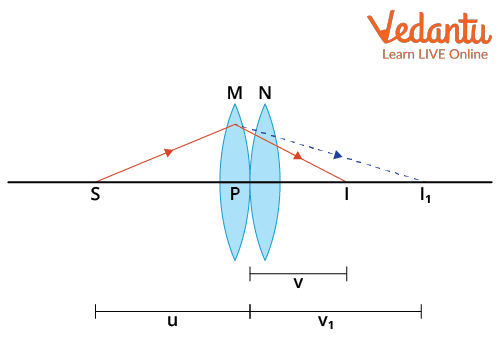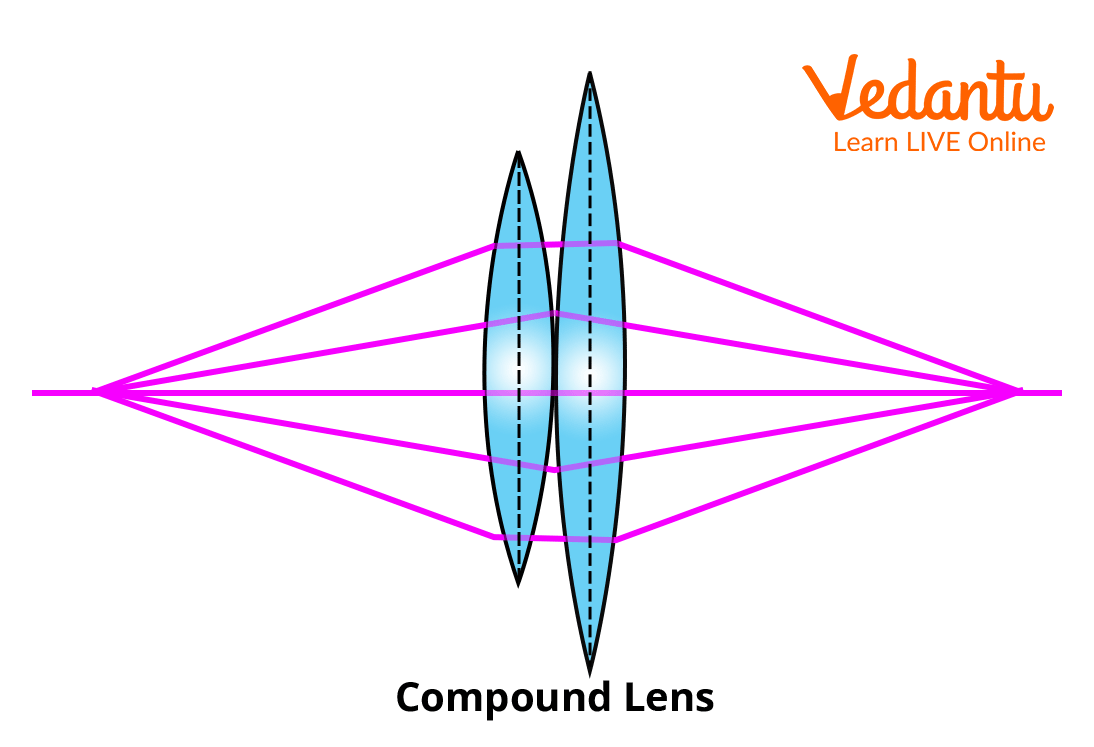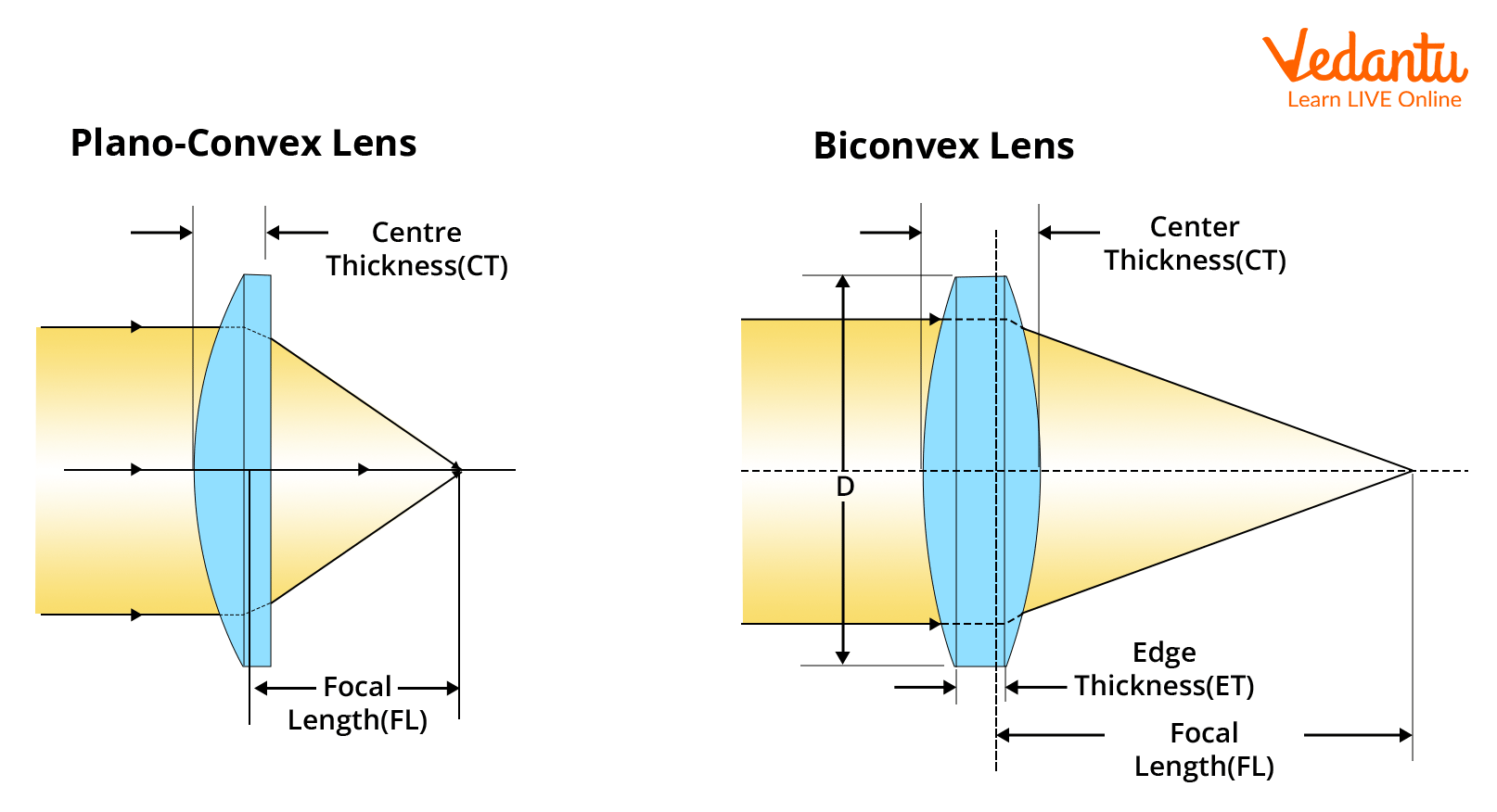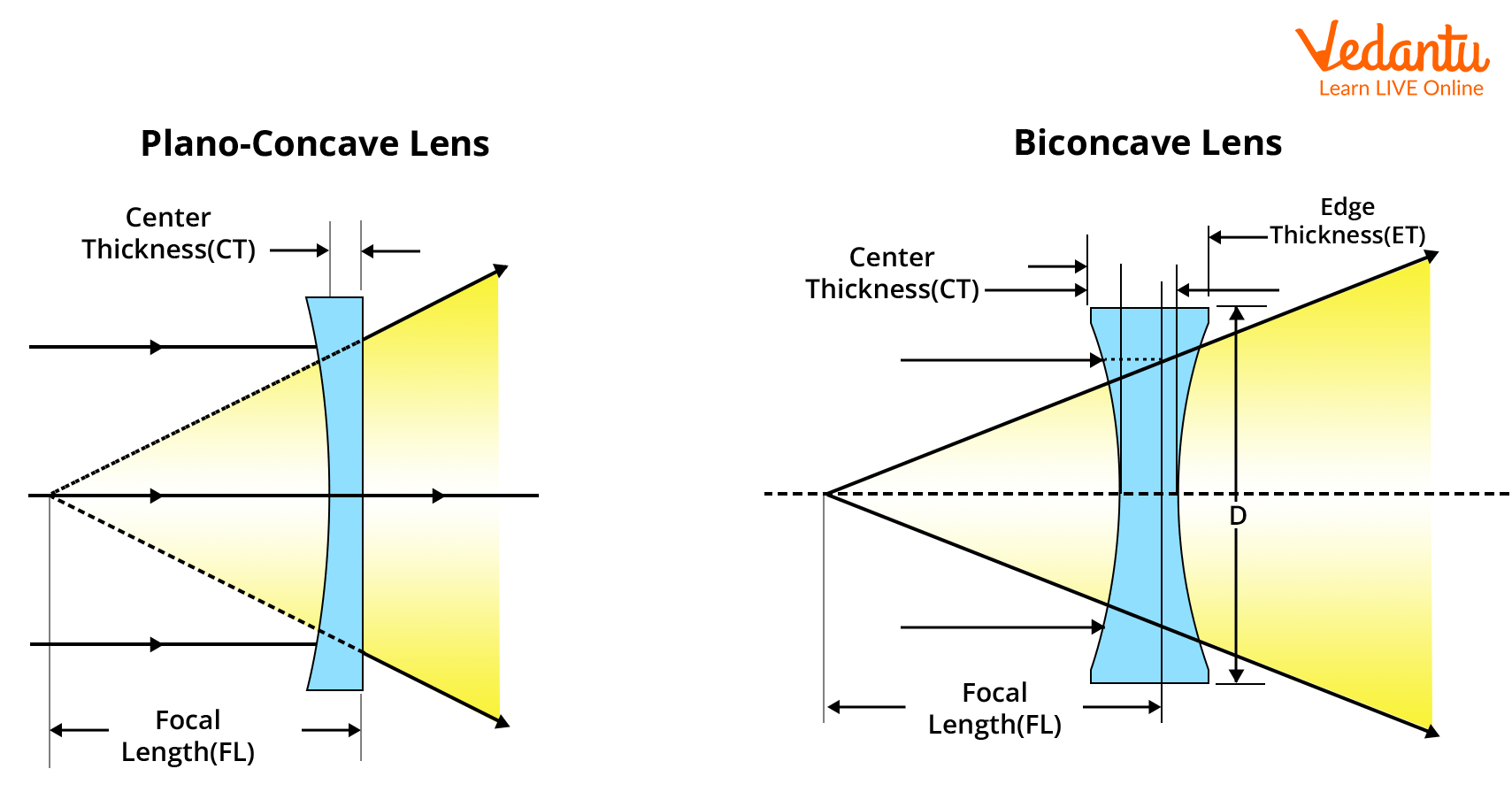Courses
Courses for Kids
Free study material
Free LIVE classes
More

# Combination of Mirrors - Formulas based Solved Examples for JEELIVE
Join Vedantu’s FREE Mastercalss

## A Brief Introduction to Combination of Mirrors

When several lenses or mirrors are combined in a co-axial direction, the image formed by the lens serves as the object for the subsequent lenses and mirrors, and the image formed by this setting serves as the object for the third lens. This is known as a combination of mirrors. When light rays from a source do not cross to form an image, a virtual image is produced by a combination of mirrors. They can instead be traced back to a point behind the lens or mirror. Multiple images are formed when an object is placed between two parallel plane mirrors that are used in conjunction.

## Combination of Lenses

Consider two lenses, M and N, with f1 and f2 focal lengths, respectively. S is positioned on the common principal axis. The first lens M produces an image at I, which becomes the object of the second lens N. The final image is created at I1, as shown in the figure. This is known as a combination of lenses.Combination of Lenses Diagram

Now, the lens formula for the image  $I_1$  produced by the first lens M is:

$\dfrac{1}{v_1} −\dfrac{ 1}{u} = \dfrac{1}{f_1}$                 …. (1)

For the second lens, N final image is formed at I.

$\dfrac{1}{v} − \dfrac{1}{v_1} =\dfrac{ 1}{f_2}$                 …. (2)

Combining equations (1) and (2),

$\dfrac{1}{v} − \dfrac{1}{u} =\dfrac{ 1}{f_1} +\dfrac{ 1}{f_2}$           …. (3)

If the combination is replaced by a single lens with a focal length F that forms an image of S at the same position I, then

$\dfrac{1}{v} − \dfrac{1}{u} =\dfrac{ 1}{F}$                       …. (4)

From equations (3) and (4),

$\dfrac{1}{F} = \dfrac{1}{f_1} +\dfrac{ 1}{f_2}$

where F is the focal length of the combination of lenses.

If there are n lenses, the equation can be written as:

$\dfrac{1}{F} = \dfrac{1}{f_1} + \dfrac{1}{f_2} + ……. + \dfrac{1}{f_n}$

Hence, this is the combination of lenses formula for focal length.

## Combination of Thin Lenses

We can examine the images formed in a lens by focusing on three main rays that were chosen for their ease of construction. All rays from the object intersect at the image point during the imaging process, so the image point can be found by finding the intersection of any two rays after they have passed through the lens. The principal rays that are typically chosen are:

(a) A ray parallel to the principal axis that must pass through the principal focus of a converging lens after refraction (or, in the case of a diverging lens, the ray must diverge from the principal focus);

(b) A ray directed towards the lens's principal focus and refracted by the lens so that it emerges parallel to the principal axis; and

(c) A ray directed towards the centre of the lens and which passes through it undeviated, because the section of the lens at its centre can be approximated as a plane parallel plate of negligible thickness.

Compound lenses are those that have two thin lenses mounted on a common axis, usually closer to each other or cemented together.Compound Lens Diagram

The following formula gives the common focal length for a system in which two thin lenses sharing an axis are kept in contact with each other.

$\dfrac{1}{f}=\dfrac{1}{f_1}+\dfrac{1}{f_2}$

where,

f is the total focal length.

The first lens' focal length is f1.

The focal length of the second lens is f2.

What is obvious in this case, given that power is the reciprocal of focal length? For thin lenses in contact, it is fairly obvious that the system's combined power is given by adding the powers of the individual lenses. But what if the lenses don't come into contact? If the lenses are separated by a distance 'd’, the combined focal length can be calculated using the formula below.

$\dfrac{1}{f}=\dfrac{1}{f_{1}}+\dfrac{1}{f_{2}}-\dfrac{d}{f_{1} \cdot f_{2}}$

## Combination of Lenses and Mirrors

The most obvious distinction between mirrors and lenses is that mirrors reflect light beams (light bounces back), whereas lenses refract (pass through) light rays. A mirror has only one point of convergence, which is directly in front of it. There are two central focuses on either side of a lens.

A concave mirror and a convex lens are similar. Light converges at the point of convergence in both a concave mirror and a raised lens. The elevated mirror and inward lens, on the other hand, effectively divide the light at the point of convergence.

When several lenses or mirrors are combined in a coaxial direction, the image formed by the lens serves as the object for the next lens or mirror, and the image formed by this setting serves as the image for the third lens. The total magnification obtained by combining a lens and a mirror is:

M = m1 $\times$ m2 $\times$ m3……..

## Plano-Convex and Biconvex Lenses

A plano-convex lens has one convex surface and one flat (planar) surface, whereas a bi-convex lens has two convex surfaces. Biconvex lenses are also used to focus light, but they work best when the imagined object is much closer to the lens. Plano-convex lenses are most commonly used in practical applications where the object being imaged is far away from the lens.Plano-Convex and Biconvex Lens Diagram

The biconvex lens formula is

$\dfrac{1}{f}=\left(\dfrac{\mu_{2}}{\mu_{1}}-1\right)\left(\dfrac{1}{R_{1}}-\dfrac{1}{R_{2}}\right)$

Here,

R1 radius of curvature of lens 1

R2 radius of curvature of lens 1

f  is the focal length of the biconvex lens

Also, light rays coming from the medium of refractive index  $\mu_2$   to the medium of refractive index $\mu_1$

The plano-convex lens formula is

For the Plano-Convex lens, we have $\mu_1=1$, $\mu_2=n$, $R_1=\infty$, $R_2=-R$.

So the focal length of the Plano-Convex lens will be as follows:

$\dfrac{1}{f}=\dfrac{n-1}{R}$

So, $f=\dfrac{R}{n-1}$

## Plano-Concave and Biconcave Lenses

A plano-concave lens is a type of optical lens that has one concave and one flat surface. Biconcave (Double-Concave) lenses have an equal radius of curvature on both sides. Plano-concave and biconcave lenses are the inverses of plano-convex and biconvex lenses. By design, the thickness of the lens at the edge is always greater than the thickness at the centre. These optics are commonly referred to as beam expanders, and the examples below show how light passing through them is dispersed from its original path.Plano-Concave and Biconcave Lens Diagram

The biconcave lens formula is:

$\dfrac{1}{f}=\left(\dfrac{\mu_{2}}{\mu_{1}}-1\right)\left(\dfrac{1}{R_{1}}-\dfrac{1}{R_{2}}\right)$

Here,

R1   radius of curvature of lens 1

R2   radius of curvature of lens 1

f  is the focal length of the biconvex lens

Also, light rays coming from the medium of refractive index $\mu_{2}$ to the medium of refractive index $\mu_{1}$

The Plano-Concave lens formula is:

For the Plano-Concave lens, we have $\mu_1=1$, $\mu_2=n$, $R_1=-R$, $R_2=-\infty$.

So the focal length of the Plano-Convex lens will be as follows:

$\dfrac{1}{f}=\dfrac{1-n}{R}$

So, $f=\dfrac{R}{1-n}$

## Solved Example on Combination of Lenses

Example 1:  If in a plano-convex lens, the radius of curvature of the convex surface is 10 cm and the focal length of the lens is 30cm, then what will be the refractive index of the material of the lens?

Solution:

As the focal length of the plano-convex lens is:

$\dfrac{1}{f}=\dfrac{n-1}{R}$

So, $f=\dfrac{R}{n-1}$

As given in the question, we have

f=20 cm, R=10 cm

So as ,

$f=\dfrac{R}{n-1}$

or

$n-1=\dfrac{R}{f}$

$n=1+\dfrac{R}{f}$

$n=1+\dfrac{10}{20}$

$n=\dfrac{3}{2}$

So the refractive index of the material of the lens will be n=1.5

Example 2: A convex lens A of focal length 10cm and a concave lens B of focal length 5cm are kept along the same axis with the distance d between them. If a parallel beam of light falling on A leaves B as a parallel beam, then what will be the distance d in cm?

Solution:

Focal length of the convex lens i.e. f1=10 cm

Focal length of the concave lens i.e.   f2=-5 cm

From the combination of lenses formula we have

$\dfrac{1}{F}=\dfrac{1}{f_{1}}+\dfrac{1}{f_{2}}-\dfrac{d}{f_{1} f_{2}}$

As the ray emerges as a parallel beam, the focal length of the combination must be the same as the plane mirror i.e., infinity. So,  $F=\infty$

By putting the values in the formula we have:

\begin{align} &\dfrac{1}{10}-\dfrac{1}{5}-\dfrac{d}{(10 \times-5)}=0 \\ &\dfrac{-d}{50}=\dfrac{1}{10}-\dfrac{1}{5} \\ &\dfrac{-d}{50}=\dfrac{1-2}{10} \\ &\dfrac{-d}{50}=-\dfrac{1}{10} \\ &d=5 \mathrm{~cm} \end{align}

Hence, distance (d) is 5 cm.

## Conclusion

In short, when several lenses or mirrors are combined in a coaxial direction, the image formed by the lens serves as the object for the next lens or mirror, and the image formed by this setting serves as the image for the third lens. This is known as a combination of mirrors. This article aids us in understanding the subject in a better way. This article thoroughly discusses the combination of lenses and mirrors and their respective formulae.

Last updated date: 18th Sep 2023
Total views: 129.3k
Views today: 2.29k

## FAQs on Combination of Mirrors - Formulas based Solved Examples for JEE

1. What characteristics distinguish an image formed by a plane mirror?

The characteristics of the image formed by a plane mirror are as follows:

• The formed image is the same size as the object.

• The plane mirror produces erect/upright and virtual images.

• The distance between the image behind the mirror and an object in front of the mirror is equal.

• One of the most important characteristics of the image formed by the plane mirror is that it is always laterally inverted. For example, if you raise your left hand, the image formed by the plane mirror will show your right hand in an upward direction.

2. What are the uses of concave mirrors and convex mirrors?

Concave mirrors are used in the following situations:

• Dentists use concave mirrors to see enlarged images of teeth.

• Concave mirrors are used to see an enlarged view of the face when applying make-up or shaving.

• Doctors (ENT specialists) use concave mirrors to focus light into the ears, throat, and other areas.

• In reflector telescopes, concave mirrors are used.

Uses of Convex Mirrors:

• Since convex mirrors increase the field of view, they are used as rear view mirrors for automobiles.

• Convex mirrors are used as street light reflectors because they distribute light more evenly.